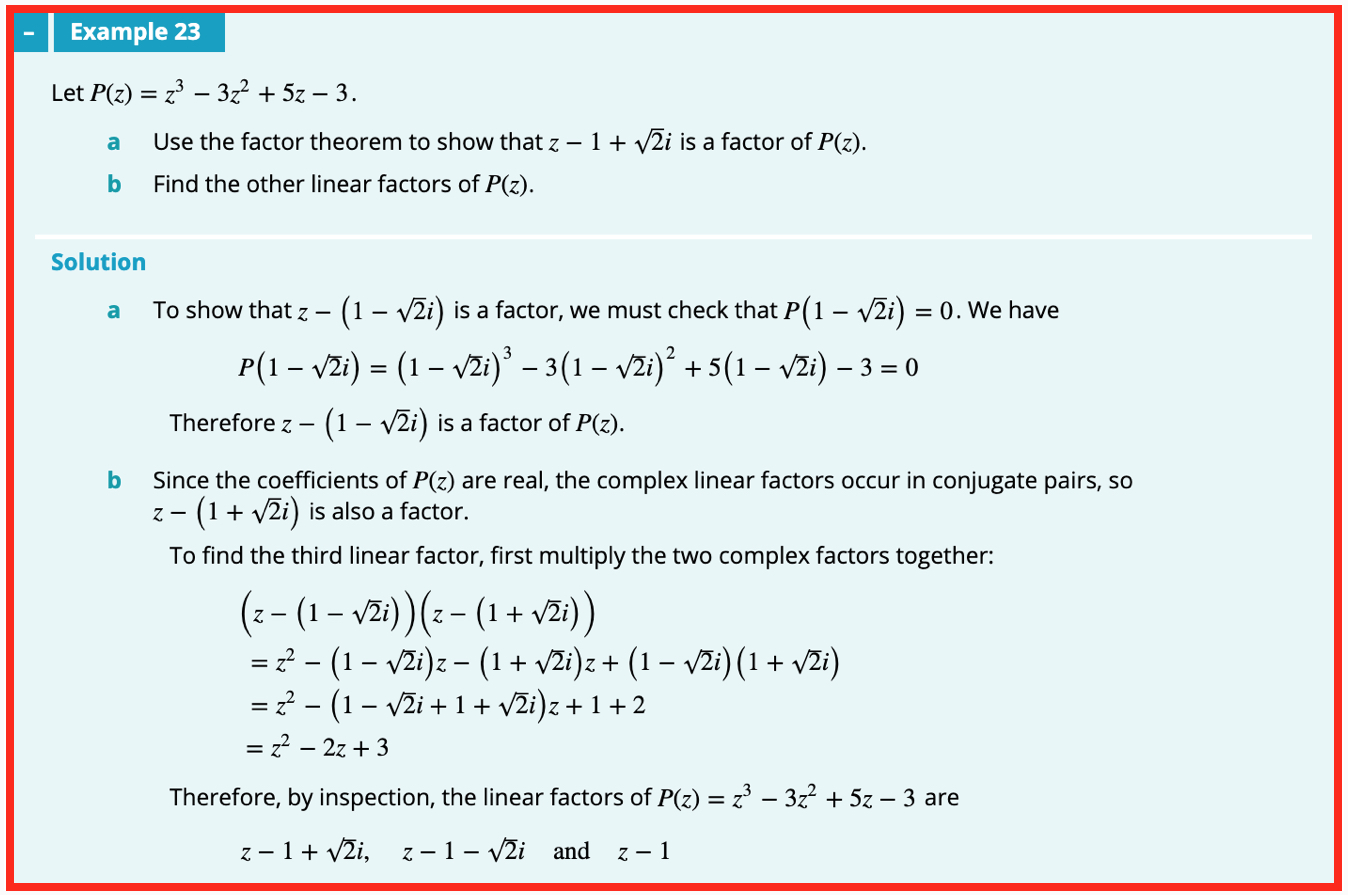## WitCH 16: The Root of Our Problem

This WitCH comes from one of our favourites, the Complex Numbers chapter from Cambridge’s Specialist Mathematics 3 & 4 (2019). It is not as deep or as beWitCHing as other aspects of the chapter. But, it’s still an impressive WitCH.## Update (11/08/19)

I guess if you’re gonna suggest a painful, ass-backwards method to solve a problem, you may as well fake the solution:

• Checking directly that P(1 – i√2) = 0 involves expanding a cubic, and more, which the text does in one single magic line.
• The painful multiplication of the products for part b is much more naturally and easily done as a difference of two squares: (z – 1 – i√2)(z – 1 + i√2) = (z – 1)2 + 2, etc.
• After all that the third factor, z – 1, is determined “by inspection”? Inspection of what?

AS RF notes, it is much easier to spot that z = 1 solves the cubic. Then some easy factoring (without long division …) gives P = (z – 1)(z2 – 2z + 3). Completing the square then leads to the linear factors, answering both parts of the question in the reverse, and natural, order.

Alternatively, as John notes, the difference of two squares calculation shows that if z – 1 + i√2 is a factor of P then so is the quadratic z^2 – 2z + 3. That this is so can then be checked (without long division …), giving P = (z – 1)(z^2 – 2z + 3), and so on, as before.